## Monday, December 24, 2012

### Segment Trees and lazy propagation

In this topic i will explain a very interesting data structure that can be used to solve a specific set of problems. I will start by explaining its definition and the proceeding with an example problem to solve with it.

• What  is segment trees?
• Order of growth of segment trees operations
• Show me your code
• Lazy propagation
• Sample problems to try
• References
What is segment trees?

Segment Trees is a Tree data structure for storing intervals, or segments, It allows querying which of the stored segments contain a given point. It is, in principle, a static structure; that is, its content cannot be modified once the structure is built. It only uses O(N lg(N)) storage.

A segment trees has only three operations: build_tree, update_tree, query_tree.

Building tree: To init the tree segments or intervals values
Update tree: To update value of an interval or segment
Query tree: To retrieve the value of an interval or segment

Example Segment Tree:
• The first node will hold the information for the interval [i, j]
• If i<j the left and right son will hold the information for the intervals [i, (i+j)/2] and [(i+j)/2+1, j]
Notice that the height of a segment tree for an interval with N elements is [logN] + 1. Here is how a segment tree for the interval [0, 9] would look like:

Order of growth of segment trees operations
• build_tree: O(N lg(N))
• update_tree: O(lg(N + k))
• query_tree: O(lg(N + k))
K = Number of retrieved intervals or segments

#### Show me your code

Lazy Propagation

Sometimes a segment tree operation wouldn't survive if the problem constraints is too large, here it come lazy propagation along with the segment tree.

In the current version when we update a range, we branch its childs even if the segment is covered within range. In the lazy version we only mark its child that it needs to be updated and update it when needed.

Note: Read my solution for problem Can you answer these queries I in this article.

Sample Problems to try
References

1.In lazy's version, i thinks it's better if you replace update tree[2*node] and tree[2*node+1] in 49th, 50th, 80th and 81th line by lazy[2*node] and lazy[2*node+1].

Its reason is your query is not really come down to higher level, so lazy[] should be updated

2.I can't understand you :)

3.So, in line 80th:
tree[node*2] += lazy[node]; // Mark child as lazy
tree[node*2+1] += lazy[node]; // Mark child as lazy

=> replaced by:
lazy[node*2] += lazy[node];
lazy[node*2+1] += lazy[node];

It's correct ?

4.Yes you are totally right :).. thanks for correcting me ;)..

5.The same at line 49 and 50, updated check it now and tell me :)

1.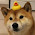2.At lines 72 and 73, shouldn't this be replaced by a lazy[node]? sorry, I am a beginner.

6.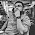update_tree(1, 0, N-1, 0, 6, 5); // Increment range [0, 6] by 5
update_tree(1, 0, N-1, 7, 10, 12); // Incremenet range [7, 10] by 12
update_tree(1, 0, N-1, 10, N-1, 100); // Increment range [10, N-1] by 100

So, The maximum element in the whole array should be 101
and your final array becomes
[6 6 6 6 6 6 6 13 13 13 13 101 101 101 101 101 101 101 101]

but your program gives output as 117 !!!

7.No it should be 113, however the size of the array needs to be (1+(1<<6)) as it should be 2^(1+lg N)..

Also there was some checks needed to be added in the lazy version.. please check it and get back to me.

8.Yes your segment tree size should be
int x = (int)(ceil(log2(N)))+1;
size = (1 << x);
This one!

9.Wow! Thanks for this post, it was very helpful! However, I'm trying to implement another update_tree_val function that sets the values from a range to one value. e.g. update_tree_val(3,7,4) would set the range [3,7] to the value of 4. How can I do this using lazy propagation on your tree?

Thanks

1.It would be the same but without incrementing..

2.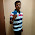This means in line 48 ,I have to modify it to tree[node] = lazy[node];
and in line 62, tree[node] = value; ?

10.This comment has been removed by the author.

11.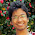in line 86 it should be
tree[node] += (b-a+1)*lazy[node];

1.I think you forgot that query returns maximum from given range
not the sum of elements of given range

2.This is true only when your query is range sum and not max range.

12.In order to do the following:
1.) Add x to A[i], A[i+1],...,A[j]
2.) Output the sum of A[i],A[i+1],...A[j]
I simply replaced max() with sum() however i am not getting the correct answer.

1.also replace line 48 and 86 by tree[node] += ( b - a + 1 ) * lazy[node];
and line 62 by tree[node] += ( b - a + 1 ) * value;

2.This comment has been removed by the author.

13.This comment has been removed by the author.

14.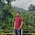in query_tree() method we could have updated the lazy value to the tree before we look for the out of range condition.... it would result in better performance...

am i right about it....please correct me if I am wrong!!

1.yes whenever u reach a node that has pending updates ..be it in the update or query function u should always update that node.this should be a good practise.

15.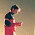Can you please elaborate how to implement DQUERY?

1.The best link is:

16.http://discuss.codechef.com/questions/50866/segment-trees-doubt

17.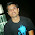18.we have to take array size of 2^n for make segment tree of n element array,then how to make segment tree of 30 or greater elements,because 2^30 = 1073741824
then how to take array of this larger size for make segment tree,how to implement ?

19.It should be 2^(1+lg N) not 2^N

20.This comment has been removed by the author.

21.Very good and nice blog..
I am following your code structure in my SG tree implementation :)

22.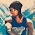This comment has been removed by the author.

23.Hey Note: Read my solution for problem Can you answer these queries I in this article.

The article doesn't point to the solution. It's pointing to the problem itself. Please correct it.

1.The link is now correct, thanks for pointing this out

24.What is the complexity of updating range
You said O(log(n+k))
But I read somewhere O(log n + k)
http://en.wikipedia.org/wiki/Segment_tree
Suppose I want to update range(0,N-1)
It works same like "build_tree" but the complexity is different

25.26.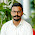Can you do range multiplication with segment trees?

1.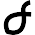of course. its same as update value with k * x (x is elements in the range)

27.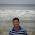How to initialize values in a range i.e A[i]=x(some integer) using update function in lazy propagation?

28.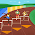If I change (2*node) with (2*node+1) and (2*node+1) with (2*node+2) and start the node position with 0 instead of 1.Will there be any changes in the result?Why have you started node of segment tree with 1?

29.in 46 line when will a > b condition becomes true?.

30.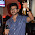well i still cant understand the lazy part.....what does the lazy[]array stores ?.......i mean for a particular node

31.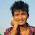I cannot say if I understood Lazy propagation part.

32.Great Explanation Of Segment trees
please explain more the lazy propagation part i cannt understand it.

33.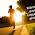i don't understand

34.thank u for your post............really helped me...........

35.If you want to learn this in a real easy way, watch tushar roy's youtube video on segment tree along with this blog post.

36.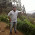D-Query can be easily solved using fast io + MO's algorithm...But using BIT is safer to avoid TLE sometimes...Nice Post...!!!

37.This comment has been removed by the author.

38.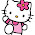39.what if i have to update each nodes with different values means i m not updating whole range with unique value

40.Wow,,, This is great blog... Thanks for sharing this informative Content here....

We offfers Tree Trimming in Sacramento, Contact us now for Tree Pruning in Sacramento

Source: Best Tree Company in Sacramento www.cisnerostreecare.com

41.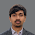nice :)

42.Hey, this is a really intuitive post with which i learnt how a segment tree is coded!
Could you point to me some links that will explain how the worst case complexity of updating intervals is O(log(N+k))?

43.The article is praiseworthy. It talks about Segment Trees and lazy propagation. The article will let you understand what are segment trees? According to the article, ‘Segment Trees is a Tree data structure for storing intervals or segments. It allows querying which of the stored segments contain a given point. A segment trees have only three operations such as build tree, update tree, and query tree.’

Thanks,
Essay writing service

44.Writing an assignment could be tough task for you for various reasons. However, our assignment writers have ample experience in writing assignments and have much time as well.You can opt for our assignment writing service and can get a well-written assignment from us

Nda admit card 2019

45.46.